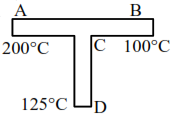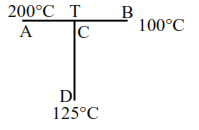# A rod CD of thermal resistance

Question:

A rod CD of thermal resistance $10.0 \mathrm{KW}^{-1}$ is joined at the middle of an identical rod $A B$ as shown in figure, The end $A, B$ and $D$ are maintained at $200^{\circ} \mathrm{C}, 100^{\circ} \mathrm{C}$ and $125^{\circ} \mathrm{C}$ respectively. The heat current in CD is $\mathrm{P}$ watt. The value of $\mathrm{P}$ is .......Solution:Rods are identical so

$R_{A B}=R_{C D}=10 \mathrm{Kw}^{-1}$

$C$ is mid-point of $A B$, so

$\mathrm{R}_{\mathrm{AC}}=\mathrm{R}_{\mathrm{CB}}=5 \mathrm{Kw}^{-1}$

at point $\mathrm{C}$

$\frac{200-\mathrm{T}}{5}=\frac{\mathrm{T}-125}{10}+\frac{\mathrm{T}-100}{5}$

$2(200-T)=T-125+2(T-100)$

$400-2 T=T-125+2 T-200$

$\mathrm{T}=\frac{725}{5}=145^{\circ} \mathrm{C}$

$\mathrm{I}_{\mathrm{h}}=\frac{145-125}{10} \mathrm{w}=\frac{20}{10} \mathrm{w}$

$\mathrm{I}_{\mathrm{h}}=2 \mathrm{~W}$